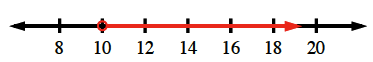### Home > CC2MN > Chapter 8 CC1: Unit 8 > Lesson CC1: 8.2.1 > Problem8-80

8-80.

Your friend, Jaime, is facing a deadline and needs your help. His English teacher told his class to read a book called The Crimson A. It has $242$ pages, and Jaime has $24$ days before he needs to be finished. He needs to figure out the minimum number of pages he needs to read each night to finish the book in time.

1. Write an inequality for Jaime’s situation. Remember to define your variable.

$24x>242$

2. Solve the inequality that your wrote in part (a). Tell Jaime how many pages he needs to read each day and graph the solution on a number line.

Solve the inequality as you would an equation.

$24x=242$ (Solve for $x$)

$x=10.0833$

Place an open circle at the solution to the equation (on the number line).
Test a number on each side to determine the direction of the arrow.

Graph the inequality on a number line.

The minimum number of pages Jaime must read each day is $11$.